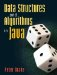# A.7. Constants

The program in Figure A-16 computes the circumference and radius of a circle. Two of the methods make use of the mathematical constant p. This program works, but it is bad style for an unidentified magic number like this to appear in several places in the code. If we have to type it several times, we might make a mistake. If we later want to change the value (for example, to specify more digits of precision), we have to find every occurrence of the number in the file. Finally, it might not be obvious to someone reading the code what this number means.

Figure A-16. The number 3.14159 appears in several places in the code. It is best to replace such a magic number with a constant.

 ``` 1 /** Compute the circumference and area of a circle. */ 2 public class Circle { 3 4 /** Return the area of a circle with the specified radius. */ 5 public static double area(double radius) { 6 return 3.14159 * radius * radius; 7 } 8 ``` 9 /** Return the circumference of a circle with the specified radius. */ 10 public static double circumference(double radius) { 11 return 3.14159 * 2 * radius; 12 } 13 14 /** 15 * Read the radius from the user and print the circumference and 16 * area. 17 */ 18 public static void main(String[] args) { 19 java.util.Scanner input = new java.util.Scanner(System.in); 20 System.out.print("Enter the radius of the circle: "); 21 double radius = input.nextDouble(); 22 System.out.println("Circumference: " + circumference(radius)); 23 System.out.println("Area: " + area(radius)); 24 } 25 26 }

A better solution is to declare a constant, a variable which cannot change. In Java, this is done with the keyword final:

```final double pi = 3.14159;
```

Where should we put this line? If we put it in circumference(), it will not be visible in area(), and vice versa. If we put it in main(), neither of the other methods will be able to see it.

The solution is to put it in the class, but not inside any particular method. Like a method, we declare it public and static (Figure A-17). A variable like this is called a class field, static field, or class variable. This is different from an instance field, which is not declared static and will be discussed in Chapter 1.

Just as we can invoke a method from another class, we can refer to a class field from another class. Two particularly useful constants appear in the built-in Math class: Math.PI (roughly 3.14159, the ratio of the circumference to the diameter of a circle) and Math.E (roughly 2.71828, the base of the natural logarithms).

We can now understand System.out.println() slightly more precisely. System is a class. There is a constant out within that class. The method println() is invoked on the object System.out. This is a nonstatic method, as will be explained in Section 1.2.

Figure A-17. The magic number has been replaced with a constant.

 ``` 1 /** Compute the circumference and area of a circle. */ 2 public class Circle { 3 4 /** Ratio of the circumference to the diameter of a circle. */ 5 public static final double PI = 3.14159; 6 7 /** Return the area of a circle with the specified radius. */ 8 public static double area(double radius) { 9 return PI * radius * radius; 10 } 11 12 /** 13 * Return the circumference of a circle with the specified 14 * radius. 15 */ 16 public static double circumference(double radius) { 17 return PI * 2 * radius; 18 } 19 20 // See Figure A-16, lines 1421, for the main() method 21 22 } ```

It is often a good idea to create a single constant instance of the java.util.Scanner class:

```public static final java.util.Scanner INPUT
= new java.util.Scanner(System.in);
```

Exercises

 A.16 Define a constant String specifying your favorite color.

### A 8 OperatorsData Structures and Algorithms in Java
ISBN: 0131469142
EAN: 2147483647
Year: 2004
Pages: 216
Authors: Peter Drake